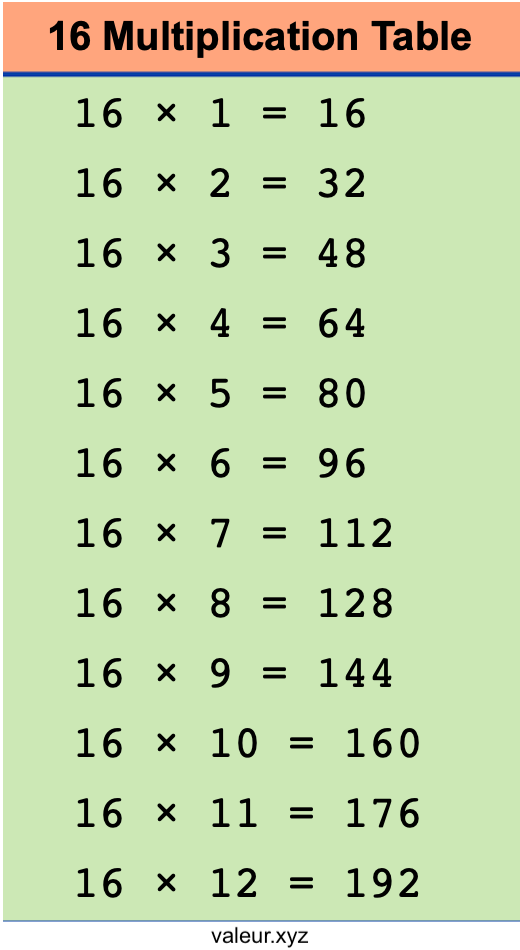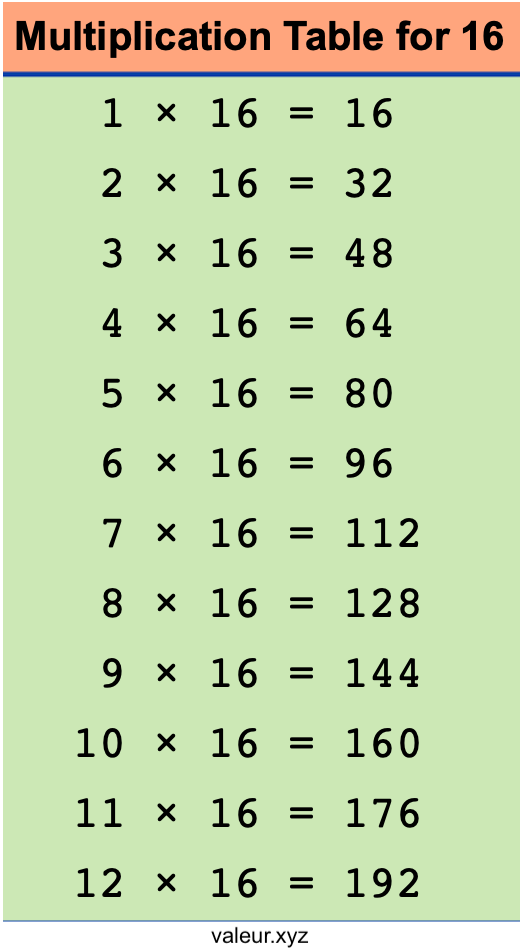16 Multiplication Table

We created a free printable multiplication table for 16 for you, so you can print it out and learn or teach someone the 16 times table.

As you can see below, we actually created two multiplication tables for 16. We made two versions of the 16 times table to illustrate that 1 times 16 is the same as 16 times 1.

Save and print the table below that you think will work best for you or your students to learn the multiplication table for 16.Multiplication Tables
Print out all of our multiplication tables! Submit the multiplication table of your choice below.

17 Multiplication Table
Here is the next multiplication table in our database that you can print out for free.